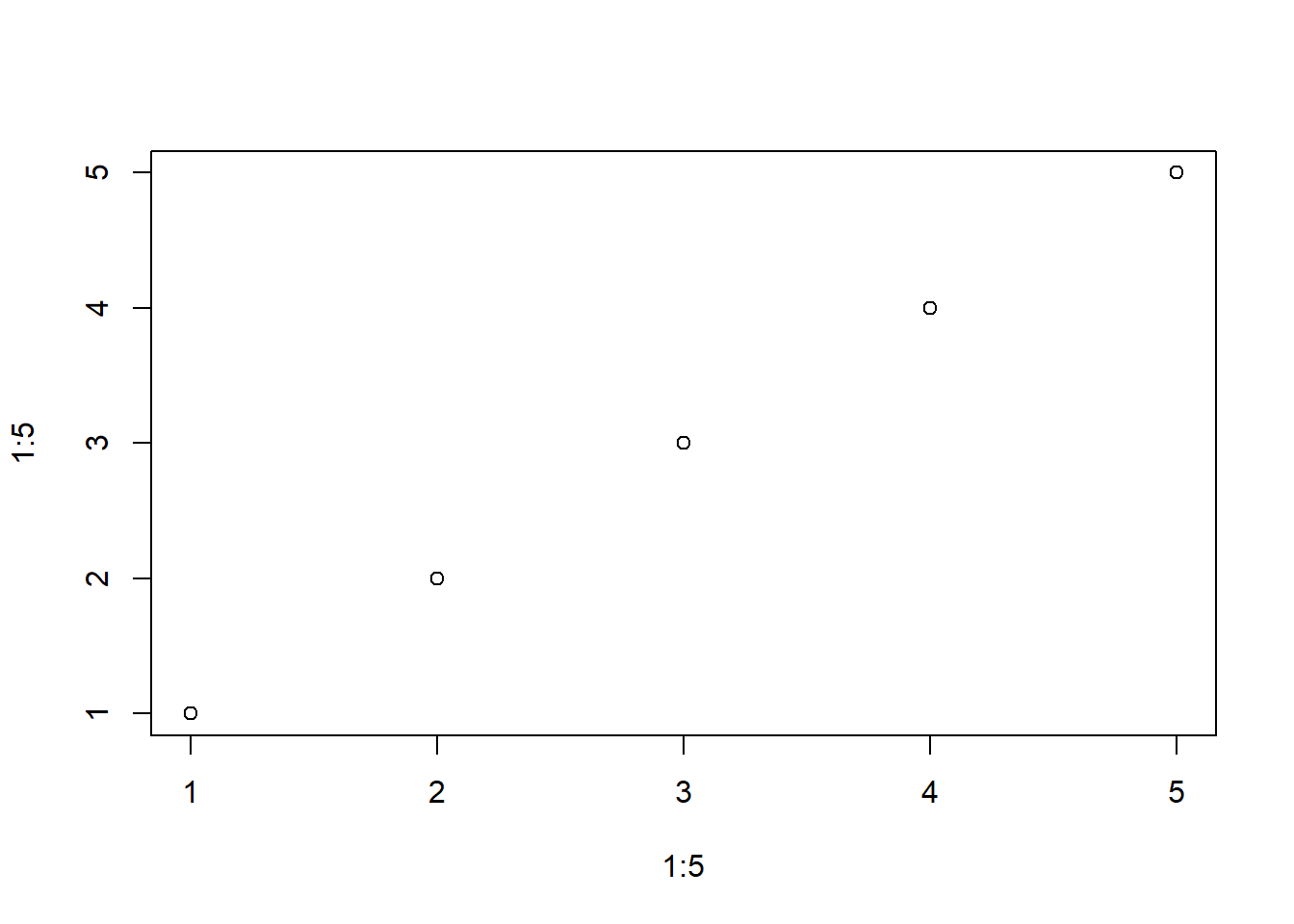# The `do.call()` function in R: Unlocking Efficiency and Flexibility

rtip
Author

Steven P. Sanderson II, MPH

Published

June 1, 2023

# Introduction

As a programmer, you’re always on the lookout for tools that can enhance your productivity and make your code more efficient. In the world of R programming, the `do.call()` function is one such gem. This often-overlooked function is a powerful tool that allows you to dynamically call other functions, opening up a world of possibilities for code organization, reusability, and flexibility. In this blog post, we will demystify the `do.call()` function in simple terms and provide you with practical examples that showcase its versatility.

# Understanding `do.call()`

At its core, the `do.call()` function in R allows you to call other functions by constructing the function call as a list. It takes two arguments: the first being the function you want to call, and the second being a list of arguments to pass to that function. By encapsulating the function call as a list, you can generate and execute function calls dynamically, making your code more adaptable and concise.

# Syntax

The syntax for the `do.call()` function is as follows:

``do.call(what, args, quote, envir)``

The parameters:

• `what` - Either a function or a non-empty character string naming the function to be called
• `args` - A list of arguments to the function call. The names attribute of args gives the argument names
• `quote` - A logical value indicating whether to quote the arguments
• `envir` - An environment within which to evaluate the call. This will be most useful if `what` is a character string and the arguments are symbols or quoted expressions

# Examples

## Example 1: Combining Multiple Vectors with `rbind()`

Let’s say you have a list of vectors, and you want to combine them into a single matrix using the rbind() function. Instead of manually specifying the vectors one by one, you can leverage do.call() to dynamically generate the function call:

``````vectors <- list(c(1, 2, 3), c(4, 5, 6), c(7, 8, 9))
combined_matrix <- do.call(rbind, vectors)
combined_matrix``````
``````     [,1] [,2] [,3]
[1,]    1    2    3
[2,]    4    5    6
[3,]    7    8    9``````

In this example, do.call() dynamically constructs the function call `rbind(c(1, 2, 3), c(4, 5, 6), c(7, 8, 9))`, resulting in a matrix that combines the vectors.

## Example 2: Applying a Function to Multiple Data Frames with `lapply()`

Suppose you have a list of data frames, and you want to apply a specific function to each of them, such as summarizing the mean of a column. Instead of writing repetitive code, you can use do.call() to apply the desired function dynamically:

``````data_frames <- list(
data.frame(a = 1:3),
data.frame(a = 4:6),
data.frame(a = 7:9)
)
mean_results <- do.call(
rbind,
lapply(data_frames, function(df) mean(df\$a))
)
mean_results``````
``````     [,1]
[1,]    2
[2,]    5
[3,]    8``````

In this example, `do.call()` combines the results of applying the mean function to each data frame’s ‘a’ column into a single matrix.

# Example 3: Dynamically Selecting and Applying Functions

One of the most powerful features of `do.call()` is the ability to dynamically select and apply functions based on runtime conditions. Consider a scenario where you have a variable number of vectors, and you want to perform different operations based on the number of vectors. `do.call()` makes it easy:

``````vectors <- list(c(1, 2, 3), c(4, 5, 6))
if (length(vectors) == 2) {
result <- do.call("+", vectors)
} else if (length(vectors) == 3) {
result <- do.call("*", vectors)
}

result``````
`` 5 7 9``

In this example, `do.call()` dynamically selects the appropriate function based on the number of vectors and applies it accordingly.

# More simple examples

``````numbers <- list(1:5)
do.call(mean, numbers)``````
`` 3``
``do.call(paste, numbers)``
`` "1" "2" "3" "4" "5"``
``do.call(sort, numbers)``
`` 1 2 3 4 5``

``````un <- unlist(numbers)
do.call(plot, list(x = un, y = un))````````````mod <- do.call(lm, list(y ~ ., data = data.frame(x = un, y = un)))
mod\$coefficients``````
`````` (Intercept)            x
1.191616e-15 1.000000e+00 ``````

# Conclusion

The `do.call()` function in R is a versatile tool that enables you to dynamically call functions, making your code more efficient and flexible. By encapsulating function calls as lists, you gain the ability to generate function calls dynamically, reducing code duplication and increasing reusability. Whether you’re combining vectors, applying functions to data frames, or dynamically selecting functions, `do.call()` empowers you to tackle complex scenarios with elegance and efficiency. Embrace the power of `do.call()` in your R programming journey, and unlock a world of possibilities!